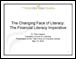# Numeracy

## Dividing Decimals - Practice [Video – 8:46] (2011)With this video tutorial, learners get the chance to practise the skills required to divide decimals.

The tutorial includes three division problems, each a little more complicated than the one before. Learners can pause the video while they work out the problems on their own, then restart it to see the instructor’s detailed solutions to the problems.

2012-05-11

## Dividing Decimals - Concept [Video – 19:52] (2011)This video introduces the concepts underlying the process of dividing with decimals.

The instructor narrating the video explains that division with decimals requires the use of long division, with special attention to the position of the decimal point.

He leads the learner through a series of problems, solving each one in a series of steps and offering tips to simplify the calculations.

2012-05-11

## Operations with Mixed Fractions Part 2- Practice [Video – 9:04] (2011)This math video tutorial offers learners a chance to practise multiplication and division with mixed fractions. A mixed fraction is one that contains both a whole number and a fraction.

The tutorial includes three math problems involving mixed fractions. Learners are asked to pause the video while they work out the problems on their own, then restart it to see the instructor’s solutions.

2012-05-03

## Operations with Mixed Fractions Part 2 – Concept [Video – 9:04] (2011)This math video tutorial introduces multiplication and division with mixed fractions.

The instructor explains the steps required, including converting a mixed fraction to an improper one; carrying out the operation; and reducing the resulting fraction to its simplest form.

He points out that with multiplication and division problems, unlike addition and subtraction ones, there is no need to find a common denominator.

2012-05-03

## Multiplying Decimals - Practice [Video – 9:39] (2011)This video tutorial offers learners the chance to practise the skills required to multiply with decimals.

It includes three problems of increasing complexity, each involving decimals. Learners are asked to pause the video while they work out the problems on their own.

Then they can restart the video and see the instructor’s detailed solutions to the problems.

2012-04-23

## Multiplying Decimals - Concept [Video – 11:46] (2011)This video tutorial offers an introduction to multiplication with decimals.

The instructor leads the learner step by step through the process of solving three problems, each one a little more challenging than the one before.

The tutorial also offers a brief review of borrowing, carrying, and the order of operations.

2012-04-23

## Adding & Subtracting Decimals – Practice [Video – 5:36] (2011)In this brief video tutorial, learners get a chance to practise their skills at adding and subtracting decimals.

The video includes three problems that illustrate a variety of challenges that may occur in calculations involving decimals. For instance, the solution to the third problem ends up being a negative number.

2012-04-17

## Adding & Subtracting Decimals - Concept [Video – 7:47] (2011)This video tutorial offers an introduction to adding and subtracting decimals.

It begins with a review of columns and their names. For instance, to the right of the decimal, the columns are called tenths, hundredths, thousandths and so on.

The instructor also touches on borrowing and carrying when performing calculations.

2012-04-17

## Marking for Confidence in Math [Video - 6:27] (2010)

Featuring Kate Nonesuch, Literacy Educator and Curriculum WriterThis video, part of a series prepared by Literacy Nova Scotia, focuses on ways to turn the marking of a math exercise into a learning situation.

2012-04-16

## The Changing Face of Literacy: The Financial Literacy Imperative (2010)

Presentation to the Task Force on Financial Literacy, May 10, 2010In this presentation to the Government of Canada’s Task Force on Financial Literacy, the president and CEO of the Canadian Council on Learning (CCL) outlines the role the CCL could play in enhancing Canadian’s financial skills.

Authors: